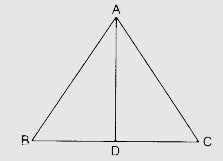# In an equilateral triangle, prove that three times the square of one side is equal to four times the square of one of its altitudes.

To do:

We have to prove that three times the square of one side of an equilateral triangle is equal to four times the square of one of its altitudes.

Solution:

Let in a $\triangle \mathrm{ABC}$,

$\mathrm{AB}=\mathrm{BC}=\mathrm{AC}$

$\mathrm{AD} \perp \mathrm{BC}$In $\triangle \mathrm{ADB}$,

$\mathrm{AB}^{2}=\mathrm{AD}^{2}+\mathrm{BD}^{2}$

$\mathrm{AD}^{2}=\mathrm{AB}^{2}-\mathrm{BD}^{2}$

$=\mathrm{AB}^{2}-(\frac{1}{2} \mathrm{BC})^{2}$

$=\mathrm{AB}^{2}-\frac{1}{4} \mathrm{BC}^{2}$

$4 \mathrm{AD}^{2}=4 \mathrm{AB}^{2}-\mathrm{BC}^{2}$

$=4 \mathrm{AB}^{2}-\mathrm{AB}^{2}$            (Since $BC=AB$)

$4 \mathrm{AD}^{2} =3 \mathrm{AB}^{2}$

Hence proved.